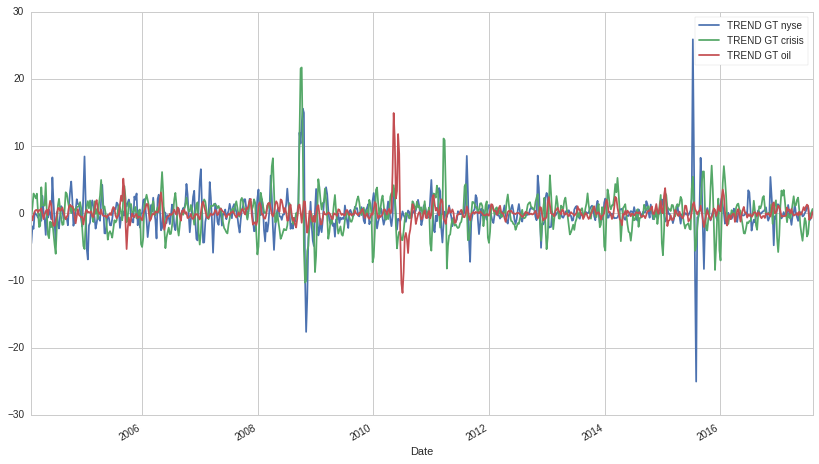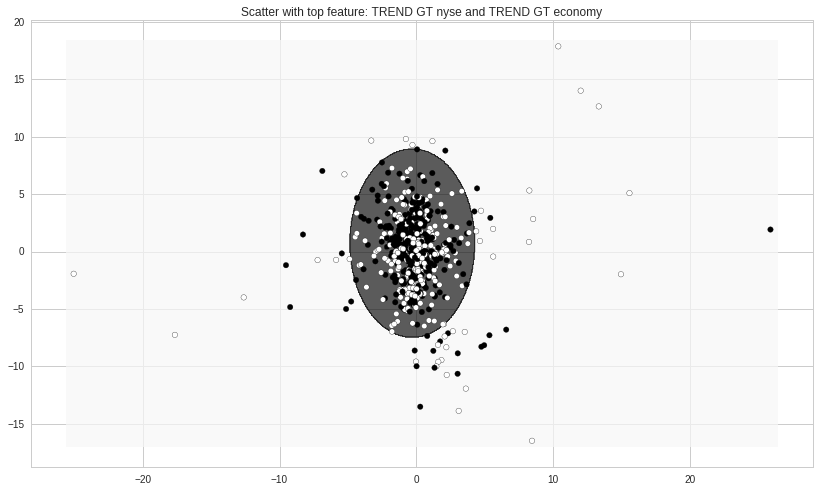Notebook

## Weekly security movement prediction using Machine Learning and Google Trends/Alternative DataÂ¶

In this notebook, I use machine learning classifiers and Google Trend data to try and predict upcoming week movement of a security. The Google Trend data includes relative search volumes for roughly 80 search terms. The search terms are all words related to a normal, everyday household conversation. Example: "crisis", "debt", "economy", "war", "china".

The idea is to see if there is some machine learnable corrolation between the public's mood and fears and the stock market.

In :
import numpy as np
import pandas as pd
from matplotlib import pyplot as plt
import datetime
from sklearn.preprocessing import MinMaxScaler
from sklearn.svm import OneClassSVM, SVC
from sklearn.naive_bayes import GaussianNB
import matplotlib.pyplot

In :
# Load pricing data for a stock or an ETF.  Other securities could be used as well.

data = get_pricing('GE', start_date='2004-01-01', end_date='2017-08-08', frequency='daily')

In :
# resample data to a weekly format.
df = pd.DataFrame()
df['open'] = data['open_price'].resample('W').first()
df['close'] = data['close_price'].resample('W').last()
df = df.shift(-1).dropna()
del data

In :
# calculate weekly gain
df['gain'] = df['close'].pct_change()

In :
def compute_result(a):
if a >= 0:
return 1
else :
return -1
df['result'] = df['gain'].apply(compute_result)

In :
# label up weeks as 1 and down weeks as -1.
data = local_csv('data.csv', date_column='Date')

In :
# merge data with google trend data.
df = pd.concat([df, data], axis=1, join='outer')
del data


The features used for the machine learning are obviously not the raw data from Google. We calculate a rolling 4 week slope. This brings the data to a stationary series as well as remove some noise.

In :
def compute_trend(a):
x = np.arange(0, len(a))
y = np.array(a)
A = np.vstack([x, np.ones(len(x))]).T
m, c = np.linalg.lstsq(A, y)
return m

In :
raw_cols = [col for col in df.columns if 'RAW ' in col]

In :
for col in raw_cols:
df[col.replace('RAW ', 'TREND ')] = df[col].rolling(window=4, min_periods=4).apply(compute_trend).shift(1)

In :
df.dropna(inplace=True)


From the plot below, we can clearly see the market crisis of 2008 and Chinese crisis of late 2015 and other events in between.

In :
df[['TREND GT nyse', 'TREND GT crisis', 'TREND GT oil']].plot()

Out:
<matplotlib.axes._subplots.AxesSubplot at 0x7f16d1bd5f90>In :
df['gain scaled'] = abs(df['gain']).reshape(-1, 1)

In :
def plot_decision_boundary(pred_func, X, Y):
x_min, x_max = X[:, 0].min() - .5, X[:, 0].max() + .5
y_min, y_max = X[:, 1].min() - .5, X[:, 1].max() + .5
h = 0.05
xx, yy = np.meshgrid(np.arange(x_min, x_max, h), np.arange(y_min, y_max, h))
Z = pred_func(np.c_[xx.ravel(), yy.ravel()])
Z = Z.reshape(xx.shape)
plt.contourf(xx, yy, Z, alpha=0.7)
plt.scatter(X[:, 0], X[:, 1], s=30, c=(Y+1)*255)

In :
features = ['TREND GT nyse', 'TREND GT economy']


Running ML on just two features (two keywords) we can see that the ML cannot classify most of the data, or at least, it classifies those weeks as "up" weeks. However, the weeks laying ouside the central zone are classified as down weeks and by the looks of it, it does a not so bad job. Clearly, this shows that when searches for certain keywords go up, and market correction follows.

In :
model = GaussianNB()
# model = OneClassSVM(nu=0.05, gamma=0.1)
# model = SVC(C=0.5, gamma=0.01)

# we can tune the Bayesian classfier to be skewed towards a up decision if required.
def modpred(X, model):
return (model.predict_proba(X)[:, 1] > 0.4)

model.fit(df[features], df['result']) #, sample_weight=df['gain scaled']) # for some reason, the Q sklearn library
# does not support the sample_weight paramter which does improve fitting.

plot_decision_boundary(lambda x: modpred(x, model), np.array(df[features]), np.array(df['result']))
# plot_decision_boundary(lambda x: model.predict(x), np.array(df[features]), np.array(df['result']))
plt.title('Scatter with top feature: ' + features + ' and ' + features)
plt.show()In :
# in sample ML score
model.score(df[features], df['result'])

Out:
0.54532577903682722
In :
# in-sample historical percent up weeks for security
((df['result'] + 1)/2).mean()

Out:
0.5155807365439093

### Forward walk test.Â¶

This is an out of sample test: each week, the classifier is trained using the previous 200 weeks of data and makes a prediction on the upcoming week.

In :
features = [f for f in df.columns if f.find('TREND GT') == 0]

model.fit(df[features], df['result']) #, sample_weight=df['gain scaled']) # for some reason, the Q sklearn library
# does not support the sample_weight paramter which does improve fitting.
model.score(df[features], df['result'])

Out:
0.57648725212464591

We use the OneClassSVM classifier in this case. It is a little more efficient then SVC or GaussianNB as it isolates the central cluster and identifies the cluster as "up" and outliers as "down". However, NB, SVC and even a feedforward NN would work.

In :
start = df.index.searchsorted(datetime.datetime(2012, 1, 1))
date_series = df.index[start:]

for d in date_series:
X = df[features]
#     model = GaussianNB()
model = OneClassSVM(nu=0.05, gamma=1.0/len(features)*2)
scaler = MinMaxScaler()
model.fit(scaler.fit_transform(X.loc[d-200:d-1, :]), df['result'][d-200:d-1], sample_weight=df['gain scaled'][d-200:d-1])
df.loc[d, 'prediction'] = model.predict(scaler.transform(X.loc[d:d, :]))


The results are ok I guess. The ML predictions would edge out a buy and hold strategy.

In :
# historical ratio of up weeks.
((df.loc[datetime.datetime(2012, 1, 1):, 'result'] + 1)/2).mean()

Out:
0.552901023890785
In :
# forward walk test result.
((df.loc[datetime.datetime(2012, 1, 1):, 'result']*df.loc[datetime.datetime(2012, 1, 1):, 'prediction'] + 1)/2).mean() # predicted ratio

Out:
0.5631399317406144